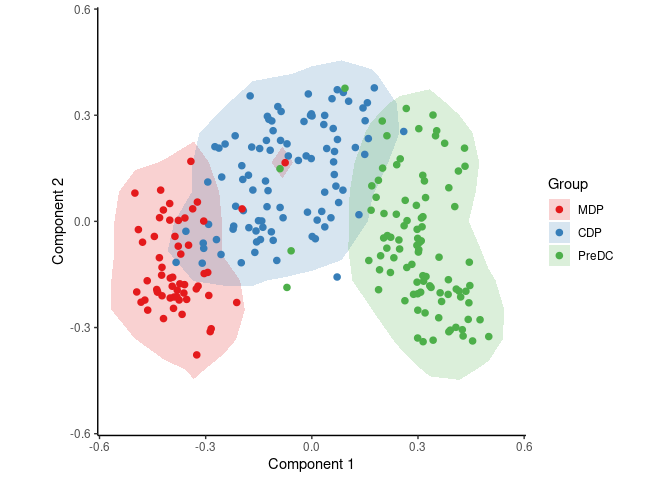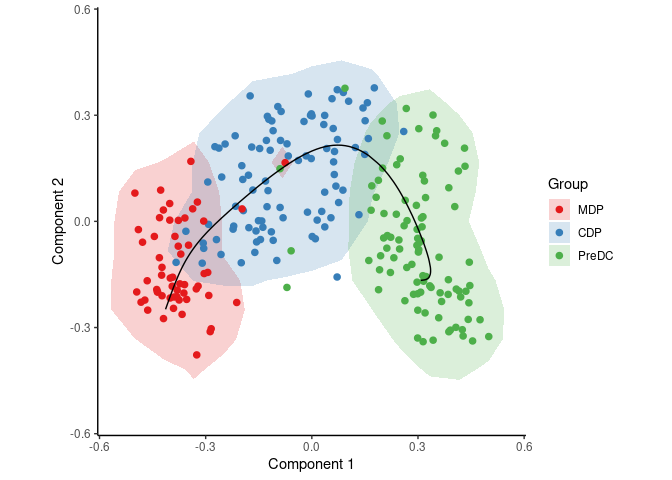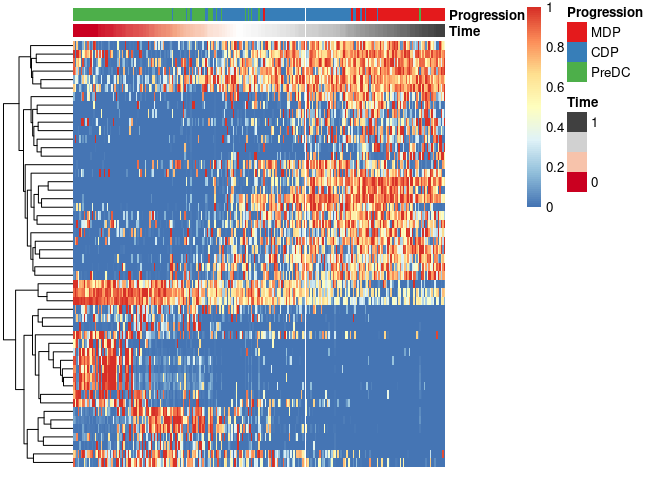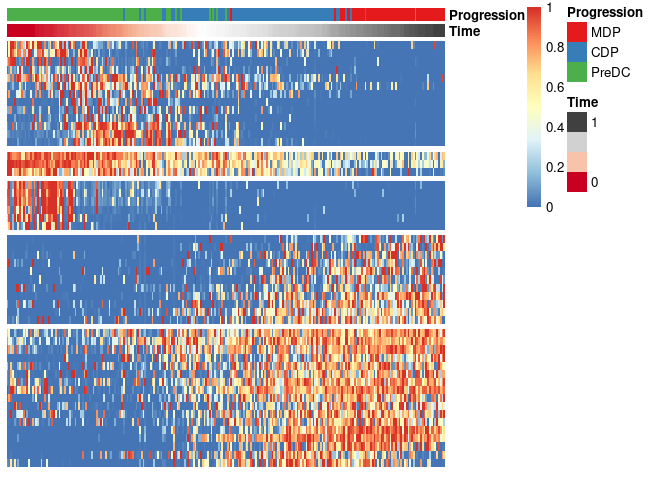# Running SCOPIUS on a Seurat object

Robrecht Cannoodt 2019-01-12

This vignette assumes that you have a Seurat object at the ready. As an example, we create one from the `ginhoux` dataset containing 248 dendritic cell progenitors.

``````library(SCORPIUS)
library(Seurat)

data(ginhoux)

counts <- t(round(2^ginhoux\$expression))
srt <- CreateSeuratObject(counts = counts, meta.data = ginhoux\$sample_info)``````

## Normalise with Seurat

Seurat is used to normalise the dataset. Since the dimensionality reduction method is scale-invariant, the scaling step in Seurat is not required.

``srt <- NormalizeData(srt)``

Fetch the expression data from the Seurat object as follows.

``expression <- t(as.matrix(srt@assays\$RNA@data))``

Also fetch some metadata from the Seurat object. Change `group_name` to whatever column in `srt@meta.data` you are interested in.

``group_name <- srt@meta.data\$group_name ``

## Reduce dimensionality of the dataset

SCORPIUS uses Torgerson multi-dimensional scaling to reduce the dataset to three dimensions. This technique attempts to place the cells in a space such that the distance between any two points in that space approximates the original distance between the two cells as well as possible.

The distance between any two samples is defined as their correlation distance, namely `1 - (cor(x, y)+1)/2`. The reduced space is constructed as follows:

``space <- reduce_dimensionality(expression, dist = "spearman", ndim = 3)``

The new space is a matrix that can be visualised with or without colouring of the different cell types.

``draw_trajectory_plot(space, progression_group = group_name, contour = TRUE)``## Inferring a trajectory through the cells

The main goal of SCORPIUS is to infer a trajectory through the cells, and orden the cells according to the inferred timeline.

SCORPIUS infers a trajectory through several intermediate steps, which are all executed as follows:

``traj <- infer_trajectory(space)``

The result is a list containing the final trajectory `path` and the inferred timeline for each sample `time`.

The trajectory can be visualised with respect to the samples by passing it to `draw_trajectory_plot`:

``````draw_trajectory_plot(
space,
progression_group = group_name,
path = traj\$path,
contour = TRUE
)``````## Finding candidate marker genes

We search for genes whose expression is seems to be a function of the trajectory timeline that was inferred, as such genes might be good candidate marker genes for dendritic cell maturation.

``````gimp <- gene_importances(expression, traj\$time, num_permutations = 0, num_threads = 8)
gene_sel <- gimp[1:50,]
expr_sel <- expression[,gene_sel\$gene]``````

To visualise the expression of the selected genes, use the `draw_trajectory_heatmap` function.

``draw_trajectory_heatmap(expr_sel, traj\$time, group_name)``Finally, these genes can also be grouped into modules as follows:

``````modules <- extract_modules(scale_quantile(expr_sel), traj\$time, verbose = FALSE)
draw_trajectory_heatmap(expr_sel, traj\$time, group_name, modules)``````